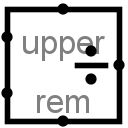#Divider

 Library: Arithmetic Introduced: 2.0 Beta 22 Appearance:## Behavior

This component divides two values coming in via the west inputs and outputs the quotient on the east output. The component is designed so that it can be cascaded with other dividers to provide support a dividend with more bits than is possible with a single divider: The upper input provides the upper dataBits bits of the dividend (if it is specified at all), and the rem bits provide the remainder, which can be fed as the upper input into another divider.

If the divisor is 0, then no division is performed (i.e., the divisor is assumed to be 1).

The divider essentially performs unsigned division. That is, the remainder will always be between 0 and divisor-1. The quotient will always be an integer so that

quotient * divisor + remainder = dividend .
If, however, the quotient does not fit into dataBits bits, then only the lower dataBits bits will be reported. The component does not provide any method for accessing the upper dataBits bits.

If either of the operands contains some floating bits or some error bits, then the component's outputs will be either entirely floating or entirely error values.

## Pins

West edge, north end:
Input:The lower dataBits bits of the dividend (that is, the first operand for the division). Bit width matches the Data Data Bits attribute.
West edge, south end:
Inout: The divisor (that is, the second operand for the division). Bit width matches the Data Data Bits attribute.
North edge: labeled upper
Inout: The upper dataBits bits of the dividend (that is, the first operand for the division). Bit width matches the Data Data Bits attribute.
East edge:
Output: The lower dataBits bits of the quotient, as specified above. Bit width matches the Data Data Bits attribute.
South edge: labeled rem
OutPut: The remainder of the division. This value will always be between 0 and divisor-1. Bit width matches the Data Data Bits attribute.

## Attributes

When the component is selected or being added, Alt-0 throught Alt-9 alter its Data Bits attribute attribute.

Data Bits
The bit width of the values to be divided and of the result.

None.

## Text Tool Behavior

None.

Back to Library Reference